# 410 - Chemistry Questions Answers

### TIPS FOR SOLID STATE

The Seven Crystal Systems

Triclinic                                                                                                                                                         The triclinic unit cell is defined by three axes, a, b, and c, of unequal lengths.None of the angles, alpha, beta, and gamma, between these axes are exactly 90°.
The standard convention for assigning axes is c < a < b.
The angle alpha lies between the b and c axes; beta lies between a and c,
and gamma lies between a and b. The cell is usually chosen
so that alpha and beta are obtuse (between 90º and 180º).

Orthorhombic
The orthorhombic unit cell is defined by three axes, a, b, and c, of unequal lengths.The angles between all axes are exactly 90°.
The axes are chosen to correspond to 2-fold axes of rotational symmetry axis
or to be perpendicular to mirror symmetry planes. The standard convention is that
c < a < b.

Hexagonal
The hexagonal unit cell can be defined by three axes. Two of them, labeled a are equalin length and at an angle of 120º to one another. The third axis, labeled c, is
perpendicular to the a axes and of a different length.
The c axis corresponds to a 3-fold or 6-fold symmetry axis.
To highlight the presence of 3-fold or 6-fold symmetry,
usual practice is to include a third a axis at 120° to the other two,
and correspondingly to use a redundant 4th integer in the Miller index.
(The extra integer is placed in the 3rd position and equals the negative of the
sum of the first two).

Trigonal
A subgroup of the hexagonal crystal system characterized by one three-fold symmetry axis.The remaining crystal systems in the hexagonal crystal system have a six-fold symmetry axis

Monoclinic
The monoclinic unit cell is defined by three axes, a, b, and c, of unequal lengths.The angles between the a and b axes and between the c and b axes are exactly 90°.
The b axis is chosen to correspond to a 2-fold axis of rotational symmetry axis
or to be perpendicular to a mirror symmetry plane.
The standard convention for assigning the other axes is c < a.
The unit cell is also chosen so that the angle beta, lying between the a and c axes
is obtuse (between 90º and 180º).

Tetragonal
The tetragonal unit cell is defined by three axes. Two of them, labeled a, are equalin length; and the c axis is of a different length. All angles between axes are 90°.
The c axis corresponds to a symmetry axis of either 4-fold rotation or
4-fold rotation inversion. The c axis can be either longer or shorter than the a axes.

Isometric, Cubic
The isometric (=cubic) unit cell is defined by three axes of equal length, all labeled a.All angles between axes are 90°. Because of the equivalence of all axes and angles.
The isometric system can contain combinations of many different types of symmetry elements:
2-, 3-, and 4- fold rotation axes, 3- and 4- fold rotation-inversion axes,
mirror planes, and centers of symmetry
Submit By: MANISH SIR 7 year ago
is this topic helpfull: 62 8

### CONCEPT OF OPTICAL ISOMERISM

OPTICAL ISOMERISM

What are isomers?

Isomers are molecules that have the same molecular formula, but have a different arrangement of the atoms in space. That excludes any different arrangements which are simply due to the molecule rotating as a whole, or rotating about particular bonds.

Where the atoms making up the various isomers are joined up in a different order, this is known as structural isomerism. Structural isomerism is not a form of stereoisomerism, and is dealt with on a separate page.

What are stereoisomers?

In stereoisomerism, the atoms making up the isomers are joined up in the same order, but still manage to have a different spatial arrangement. Optical isomerism is one form of stereoisomerism.

Optical isomerism

Why optical isomers?

Optical isomers are named like this because of their effect on plane polarised light.

Simple substances which show optical isomerism exist as two isomers known as enantiomers.

• A solution of one enantiomer rotates the plane of polarisation in a clockwise direction. This enantiomer is known as the (+)form.

For example, one of the optical isomers (enantiomers) of the amino acid alanine is known as (+)alanine.

• A solution of the other enantiomer rotates the plane of polarisation in an anti-clockwise direction. This enantiomer is known as the (-) form. So the other enantiomer of alanine is known as or (-)alanine.

• If the solutions are equally concentrated the amount of rotation caused by the two isomers is exactly the same - but in opposite directions.

• When optically active substances are made in the lab, they often occur as a 50/50 mixture of the two enantiomers. This is known as a racemic mixture or racemate. It has no effect on plane polarised light.

How optical isomers arise

The examples of organic optical isomers required at A' level all contain a carbon atom joined to four different groups. These two models each have the same groups joined to the central carbon atom, but still manage to be different: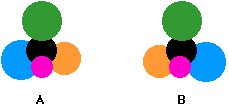Obviously as they are drawn, the orange and blue groups aren't aligned the same way. Could you get them to align by rotating one of the molecules? The next diagram shows what happens if you rotate molecule B.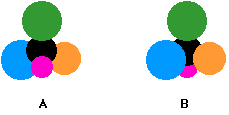They still aren't the same - and there is no way that you can rotate them so that they look exactly the same. These are isomers of each other.

They are described as being non-superimposable in the sense that (if you imagine molecule B being turned into a ghostly version of itself) you couldn't slide one molecule exactly over the other one. Something would always be pointing in the wrong direction.

What happens if two of the groups attached to the central carbon atom are the same? The next diagram shows this possibility.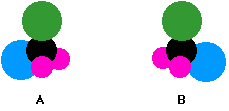The two models are aligned exactly as before, but the orange group has been replaced by another pink one.

Rotating molecule B this time shows that it is exactly the same as molecule A. You only get optical isomers if all four groups attached to the central carbon are different.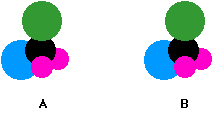Chiral and achiral molecules

The essential difference between the two examples we've looked at lies in the symmetry of the molecules.

If there are two groups the same attached to the central carbon atom, the molecule has a plane of symmetry. If you imagine slicing through the molecule, the left-hand side is an exact reflection of the right-hand side.

Where there are four groups attached, there is no symmetry anywhere in the molecule.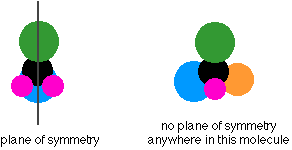A molecule which has no plane of symmetry is described aschiral. The carbon atom with the four different groups attached which causes this lack of symmetry is described as a chiral centre or as an asymmetric carbon atom.

The molecule on the left above (with a plane of symmetry) is described as achiral.

Only chiral molecules have optical isomers.

The relationship between the enantiomers

One of the enantiomers is simply a non-superimposable mirror image of the other one.

In other words, if one isomer looked in a mirror, what it would see is the other one. The two isomers (the original one and its mirror image) have a different spatial arrangement, and so can't be superimposed on each other.If an achiral molecule (one with a plane of symmetry) looked in a mirror, you would always find that by rotating the image in space, you could make the two look identical. It would be possible to superimpose the original molecule and its mirror image.

Some real examples of optical isomers

Butan-2-ol

The asymmetric carbon atom in a compound (the one with four different groups attached) is often shown by a star.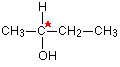It's extremely important to draw the isomers correctly. Draw one of them using standard bond notation to show the 3-dimensional arrangement around the asymmetric carbon atom. Then draw the mirror to show the examiner that you know what you are doing, and then the mirror image.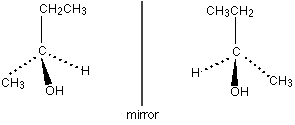Notice that you don't literally draw the mirror images of all the letters and numbers! It is, however, quite useful to reverse large groups - look, for example, at the ethyl group at the top of the diagram.

It doesn't matter in the least in what order you draw the four groups around the central carbon. As long as your mirror image is drawn accurately, you will automatically have drawn the two isomers.

So which of these two isomers is (+)butan-2-ol and which is (-)butan-2-ol? There is no simple way of telling that. For A'level purposes, you can just ignore that problem - all you need to be able to do is to draw the two isomers correctly.

2-hydroxypropanoic acid (lactic acid)

Once again the chiral centre is shown by a star.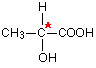The two enantiomers are: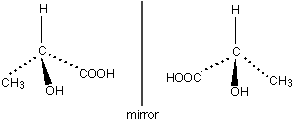It is important this time to draw the COOH group backwards in the mirror image. If you don't there is a good chance of you joining it on to the central carbon wrongly.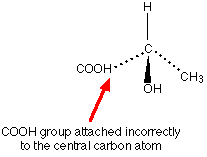If you draw it like this in an exam, you won't get the mark for that isomer even if you have drawn everything else perfectly.

2-aminopropanoic acid (alanine)

This is typical of naturally-occurring amino acids. Structurally, it is just like the last example, except that the -OH group is replaced by -NH2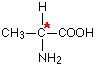The two enantiomers are: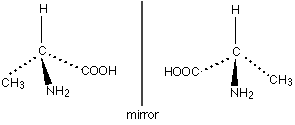Only one of these isomers occurs naturally: the (+) form. You can't tell just by looking at the structures which this is.

It has, however, been possible to work out which of these structures is which. Naturally occurring alanine is the right-hand structure, and the way the groups are arranged around the central carbon atom is known as an L- configuration. Notice the use of thecapital L. The other configuration is known as D-.

So you may well find alanine described as L-(+)alanine.

That means that it has this particular structure and rotates the plane of polarisation clockwise.

Even if you know that a different compound has an arrangement of groups similar to alanine, you still can't say which way it will rotate the plane of polarisation.

The other amino acids, for example, have the same arrangement of groups as alanine does (all that changes is the CH3 group), but some are (+) forms and others are (-) forms.

It's quite common for natural systems to only work with one of the enantiomers of an optically active substance. It isn't too difficult to see why that might be. Because the molecules have different spatial arrangements of their various groups, only one of them is likely to fit properly into the active sites on the enzymes they work with.

In the lab, it is quite common to produce equal amounts of both forms of a compound when it is synthesised. This happens just by chance, and you tend to get racemic mixtures.

Identifying chiral centres in skeletal formulae

A skeletal formula is the most stripped-down formula possible. Look at the structural formula and skeletal formula for butan-2-ol.Notice that in the skeletal formula all of the carbon atoms have been left out, as well as all of the hydrogen atoms attached to carbons.

In a skeletal diagram of this sort:

• there is a carbon atom at each junction between bonds in a chain and at the end of each bond (unless there is something else there already - like the -OH group in the example);

• there are enough hydrogen atoms attached to each carbon to make the total number of bonds on that carbon up to 4.

We have already discussed the butan-2-ol case further up the page, and you know that it has optical isomers. The second carbon atom (the one with the -OH attached) has four different groups around it, and so is a chiral centre.

Is this obvious from the skeletal formula?

Well, it is, provided you remember that each carbon atom has to have 4 bonds going away from it. Since the second carbon here only seems to have 3, there must also be a hydrogen attached to that carbon. So it has a hydrogen, an -OH group, and two different hydrocarbon groups (methyl and ethyl).

Four different groups around a carbon atom means that it is a chiral centre.

A slightly more complicated case: 2,3-dimethylpentane

The diagrams show an uncluttered skeletal formula, and a repeat of it with two of the carbons labelled.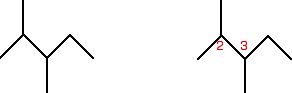Look first at the carbon atom labelled 2. Is this a chiral centre?

No, it isn't. Two bonds (one vertical and one to the left) are both attached to methyl groups. In addition, of course, there is a hydrogen atom and the more complicated hydrocarbon group to the right. It doesn't have 4 different groups attached, and so isn't a chiral centre.

What about the number 3 carbon atom?

This has a methyl group below it, an ethyl group to the right, and a more complicated hydrocarbon group to the left. Plus, of course, a hydrogen atom to make up the 4 bonds that have to be formed by the carbon. That means that it is attached to 4 different things, and so is a chiral centre.

Introducing rings - further complications

At the time of writing, one of the UK-based exam boards (Cambridge International - CIE) commonly asked about the number of chiral centres in some very complicated molecules involving rings of carbon atoms. The rest of this page is to teach you how to cope with these.When you are looking at rings like this, as far as optical isomerism is concerned, you don't need to look at any carbon in a double bond. You also don't need to look at any junction which only has two bonds going away from it. In that case, there must be 2 hydrogens attached, and so there can't possibly be 4 different groups attached.

In this case, that means that you only need to look at the carbon with the -OH group attached.

It has an -OH group, a hydrogen (to make up the total number of bonds to four), and links to two carbon atoms. How does the fact that these carbon atoms are part of a ring affect things?

You just need to trace back around the ring from both sides of the carbon you are looking at. Is the arrangement in both directionsexactly the same? In this case, it isn't. Going in one direction, you come immediately to a carbon with a double bond. In the other direction, you meet two singly bonded carbon atoms, and then one with a double bond.

That means that you haven't got two identical hydrocarbon groups attached to the carbon you are interested in, and so it has 4 different groups in total around it. It is asymmetric - a chiral centre.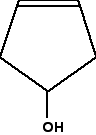In this case, everything is as before, except that if you trace around the ring clockwise and anticlockwise from the carbon at the bottom of the ring, there is an identical pattern in both directions. You can think of the bottom carbon being attached to a hydrogen, an -OH group, and two identical hydrocarbon groups.

It therefore isn't a chiral centre.

The other thing which is very noticeable about this molecule is that there is a plane of symmetry through the carbon atom we are interested in. If you chopped it in half through this carbon, one side of the molecule would be an exact reflection of the other. In the first ring molecule above, that isn't the case.

If you can see a plane of symmetry through the carbon atom it won't be a chiral centre. If there isn't a plane of symmetry, it will be a chiral centre.

A seriously complicated example - cholesterol

The skeletal diagram shows the structure of cholesterol. Some of the carbon atoms have been numbered for discussion purposes below. These are not part of the normal system for numbering the carbon atoms in cholesterol.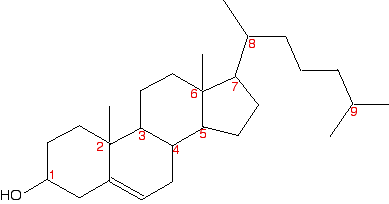Before you read on, look carefully at each of the numbered carbon atoms, and decide which of them are chiral centres. The other carbon atoms in the structure can't be chiral centres, because they are either parts of double bonds, or are joined to either two or three hydrogen atoms.

So . . . how many chiral centres did you find? In fact, there are 8 chiral centres out of the total of 9 carbons marked. If you didn't find all eight, go back and have another look before you read any further. It might help to sketch the structure on a piece of paper and draw in any missing hydrogens attached to the numbered carbons, and write in the methyl groups at the end of the branches as well.

This is done for you below, but it would be a lot better if you did it yourself and then checked your sketch afterwards.Starting with the easy one - it is obvious that carbon 9 has two methyl groups attached. It doesn't have 4 different groups, and so can't be chiral.

If you take a general look at the rest, it is fairly clear that none of them has a plane of symmetry through the numbered carbons. Therefore they are all likely to be chiral centres. But it's worth checking to see what is attached to each of them.

Carbon 1 has a hydrogen, an -OH and two different hydrocarbon chains (actually bits of rings) attached. Check clockwise and anticlockwise, and you will see that the arrangement isn't identical in each direction. Four different groups means a chiral centre.

Carbon 2 has a methyl and three other different hydrocarbon groups. If you check along all three bits of rings , they are all different - another chiral centre. This is also true of carbon 6.

Carbons 3, 4, 5 and 7 are all basically the same. Each is attached to a hydrogen and three different bits of rings. All of these are chiral centres.

Finally, carbon 8 has a hydrogen, a methyl group, and two different hydrocarbon groups attached. Again, this is a chiral centre.

This all looks difficult at first glance, but it isn't. You do, however, have to take a great deal of care in working through it - it is amazingly easy to miss one out.

Submit By: MANISH SIR 7 year ago
is this topic helpfull: 29 0

how to calculTE RESONANCE ENERGY OF CO2 IF U R GIVEN WITH

ENTHALPY OF ATOMISATION OF GRAPHITE

ENTHALPY PF FORMATION OF GRAPHITE

BE OF CO BOND

BE OF O2 BOND

Asked By: ANIKET 8 Month ago
Asked By: ANSHULA SETH 9 Month ago

Asked By: ANSHULA SETH 9 Month ago

HA is a weak acid . the ph of 0.1 M HA solution is 2 . What is the degree of dissociation of HA ?

Asked By: MUSKAN 10 Month ago

When 1 g of anhydrous oxalic acid is burnt at 25 °C, the amount of heat liberated is 2.835 kJ. ΔH combustion is (Oxalic acid : C2H2O4)

Asked By: RAJ 10 Month ago

The freezing point of 0.2 molal urea solution is -0.36 C.The freezing point of 0.02 molal solution of acetic acid is -0.0378 C.Ten the Ph os acetic acid solution is(assume molality=molarity)

Asked By: MATHEW PAUL V 11 Month ago

20 ml of 0.5M hcl is mixed with 30ml of 0.3Mhcl  . xmolarity ?

Asked By: SIDDHARTHINI 1 year ago
Asked By: SIDDHARTHINI 1 year ago

volume of gas ntp required to completely burn 1kg of coal(100% carbon) is

Asked By: YASH GOVIL 1 year ago